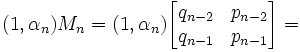Wednesday, January 04, 2006

Continued Fractions and Matrices Continued

Today's blog continues a previous blog on how 2x2 matrices can be used to represent continued fractions. The lemmas presented in today's blog are used in the solution to Pell's Equation. For those interested in the background to Pell's Equation, start here. For those who would like to proceed to its solution using continued fractions and matrices, go here. The solution to Pell's Equation is used as part of the proof for Fermat's Last Theorem, n=5.

The content in today's blog is based on Harold M. Stark's An Introduction to Number Theory.

For Lemma 1, αn, qn are defined here. For Lemma 2, Mn is defined here.

Lemma 1: αnqn-1 + qn-2 ≠ 0

(1) Assume that αnqn-1 + qn-2 = 0.

(2) Then, qn-1 = 0 and qn-2 = 0. [See here for proof]

(3) But qn ≥ 1 for n ≥ 1. [See here]

(4) Futher, there are no successive 0's in a row since (see here for details on how these values are derived):
q-2 = 1
q-1=0
q0=1

(5) Therefore #2 is impossible and we reject our assumption.

QED

Lemma 2: if γn = αnqn-1 + qn-2, then γn(1,α) = (1,αn)Mn

(1) From the definition of Mn (see here), we know that:= (qn-2nqn-1, pn-2npn-1) [See here for review on matrix products]

(2) By applying the equation α = (pn-2 + αnpn-1)/(qn-2 + αnqn-1) [See here], we get:

(qn-2nqn-1 , pn-2npn-1) = (qn-2 + αnqn-1)(1,α)

(3) Adding back γn, this gives us:
(qn-2 + αnqn-1)(1,α) = γn(1,α)

QED

Lemma 3: if δ = γn+jn, then δ(1,√d) = (1,√d)Mn-1Mn+kj

(1) From Lemma 2, we know that;

γn(1 , α) = (1, αn)Mn

and

γn+j(1, α) = (1, αn+j)Mn+j

(2) By the definition of matrix inverses (see here), we know that:
(1,αn)MnMn-1 = (1,αn)

(3) From #1, then we have:
[(1,αn)Mn)] = γn(1,α)

(4) So, from #2, we have:
(1,αn) = γn(1,α)Mn-1

(5) Likewise since αn = αn+j (see here), we get:
(1,αn+j)Mn+j = (1,αn)Mn+j

(6) Applying #4, this gives us:
(1,αn+j)Mn+j = γn(1,α)Mn-1Mn+j

(7) Combining #1 with #6, gives us:
γn+j(1,α) = γn(1,α)Mn-1Mn+j

(8) Now, δ = γn+jn [We can do this since γn ≠ 0 (see Lemma 1 above) ]

(9) δ(1,α) = [(γn+j)(1 , α)]/γn

(10) Applying #7 gives us;

δ(1,α) = [γn(1,α)Mn-1Mn+j]/γn =
= (1,α)Mn-1Mn+j

QED